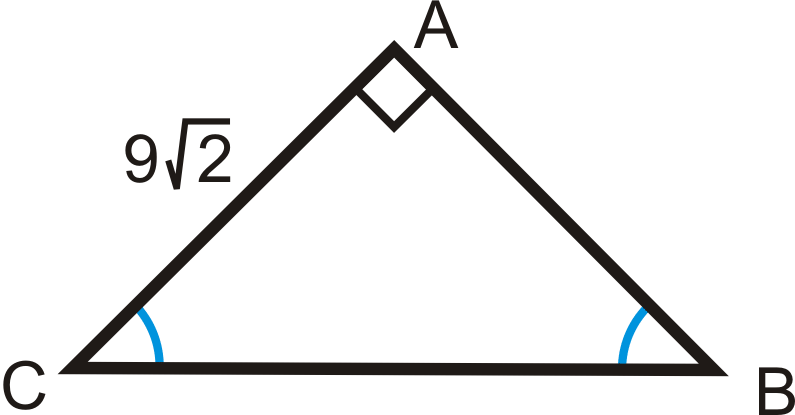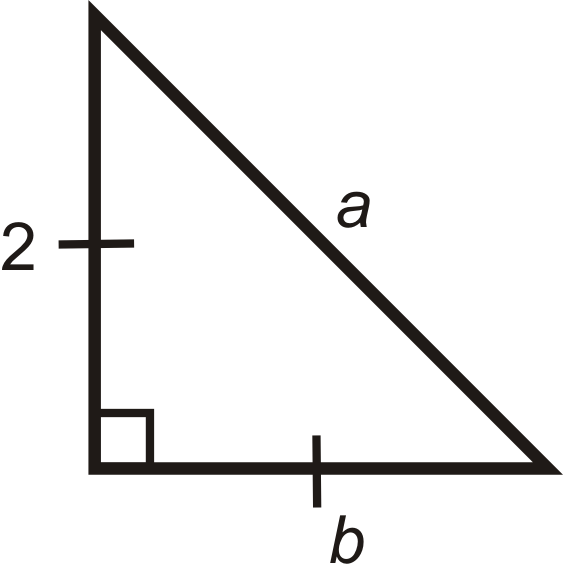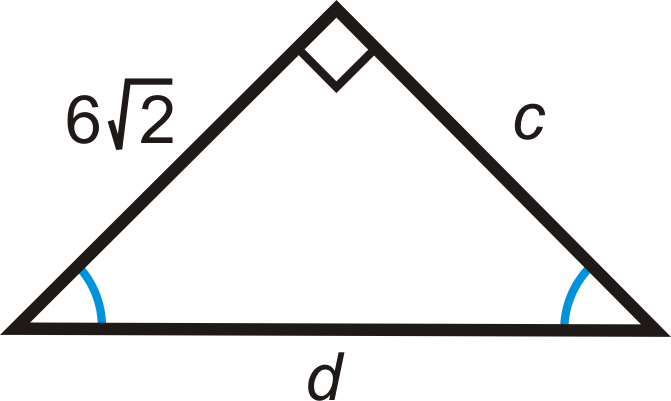# 4.42: 45-45-90 Right Triangles

•• Contributed by CK12
• CK12

Leg times $$\sqrt{2}$$ equals hypotenuse.

A right triangle with congruent legs and acute angles is an Isosceles Right Triangle. This triangle is also called a 45-45-90 triangle (named after the angle measures).Figure $$\PageIndex{1}$$

$$\Delta ABC$$ is a right triangle with $$m\angle A=90^{\circ}$$, $$\overline{AB}\cong \overline{AC}$$ and $$m\angle B=m\angle C=45^{\circ}$$.

45-45-90 Theorem: If a right triangle is isosceles, then its sides are in the ratio $$x:x:x\sqrt{2}$$. For any isosceles right triangle, the legs are x and the hypotenuse is always $$x\sqrt{2}$$.

What if you were given an isosceles right triangle and the length of one of its sides? How could you figure out the lengths of its other sides?

Example $$\PageIndex{1}$$

Find the length of $$x$$.Figure $$\PageIndex{2}$$

Solution

Use the $$x:x:x\sqrt{2}$$ ratio.

Here, we are given the hypotenuse. Solve for $$x$$ in the ratio.

\begin{aligned} x\sqrt{2}&=16 \\ x&=\dfrac{16}{\sqrt{2}}\cdot \dfrac{\sqrt{2}}{\sqrt{2}}=\dfrac{16\sqrt{2}}{2}=8\sqrt{2}\end{aligned}

Example $$\PageIndex{2}$$

Find the length of $$x$$, where $$x$$ is the hypotenuse of a 45-45-90 triangle with leg lengths of $$5\sqrt{3}$$.

Solution

Use the $$x:x:x\sqrt{2}$$ ratio.

$$x=5\sqrt{3}\cdot \sqrt{2}=5\sqrt{6}$$

Example $$\PageIndex{3}$$

Find the length of the missing side.Figure $$\PageIndex{3}$$

Solution

Use the $$x:x:x\sqrt{2}$$ ratio. $$TV=6$$ because it is equal to $$ST$$. So, $$SV=6\cdot \sqrt{2}=6\sqrt{2}$$.

Example $$\PageIndex{4}$$

Find the length of the missing side.Figure $$\PageIndex{4}$$

Solution

Use the $$x:x:x\sqrt{2}$$ ratio. $$AB=9\sqrt{2}$$ because it is equal to $$AC$$. So, $$BC=9\sqrt{2}\cdot \sqrt{2}=9\cdot 2=18$$.

Example $$\PageIndex{5}$$

A square has a diagonal with length 10, what are the lengths of the sides?

SolutionFigure $$\PageIndex{5}$$

We know half of a square is a 45-45-90 triangle, so $$10=s\sqrt{2}$$.

\begin{aligned} s\sqrt{2}&=10 \\ s&=10\sqrt{2}\cdot \sqrt{2}\sqrt{2}=10\sqrt{2}2=5\sqrt{2}\end{aligned}

## Review

1. In an isosceles right triangle, if a leg is 4, then the hypotenuse is __________.
2. In an isosceles right triangle, if a leg is $$x$$, then the hypotenuse is __________.
3. A square has sides of length 15. What is the length of the diagonal?
4. A square’s diagonal is 22. What is the length of each side?

For questions 5-11, find the lengths of the missing sides. Simplify all radicals.

1.Figure $$\PageIndex{6}$$
2.Figure $$\PageIndex{7}$$
3.Figure $$\PageIndex{8}$$
4.Figure $$\PageIndex{9}$$
5.Figure $$\PageIndex{10}$$
6.Figure $$\PageIndex{11}$$
7.Figure $$\PageIndex{12}$$

## Vocabulary

Term Definition
45-45-90 Theorem For any isosceles right triangle, if the legs are x units long, the hypotenuse is always $$x\sqrt{2}$$.
45-45-90 Triangle A 45-45-90 triangle is a special right triangle with angles of $$45^{\circ}$$, $$45^{\circ}$$, and $$90^{\circ}$$.
Hypotenuse The hypotenuse of a right triangle is the longest side of the right triangle. It is across from the right angle.
Legs of a Right Triangle The legs of a right triangle are the two shorter sides of the right triangle. Legs are adjacent to the right angle.
Radical The $$\sqrt$$, or square root, sign.

Interactive Element

Video: Solving Special Right Triangles

Activities: 45-45-90 Right Triangles Discussion Questions

Study Aids: Special Right Triangles Study Guide

Practice: 45-45-90 Right Triangles

Real World: Fighting the War on Drugs Using Geometry and Special Triangles